# gr.pygr.mlab Reference¶

This module offers a simple, matlab-style API built on top of the gr package.

## Output Functions¶

### Line Plots¶

gr.pygr.mlab.plot(*args, **kwargs)[source]

Draw one or more line plots.

This function can receive one or more of the following:

• x values and y values, or
• x values and a callable to determine y values, or
• y values only, with their indices as x values
Parameters: args – the data to plot

Usage examples:

>>> # Create example data
>>> x = np.linspace(-2, 2, 40)
>>> y = 2*x+4
>>> # Plot x and y
>>> mlab.plot(x, y)
>>> # Plot x and a callable
>>> mlab.plot(x, lambda x: x: x**3 + x**2 + x)
>>> # Plot y, using its indices for the x values
>>> mlab.plot(y)gr.pygr.mlab.oplot(*args, **kwargs)[source]

Draw one or more line plots over another plot.

This function can receive one or more of the following:

• x values and y values, or
• x values and a callable to determine y values, or
• y values only, with their indices as x values
Parameters: args – the data to plot

Usage examples:

>>> # Create example data
>>> x = np.linspace(-2, 2, 40)
>>> y = 2*x+4
>>> # Draw the first plot
>>> mlab.plot(x, y)
>>> # Plot graph over it
>>> mlab.oplot(x, lambda x: x**3 + x**2 + x)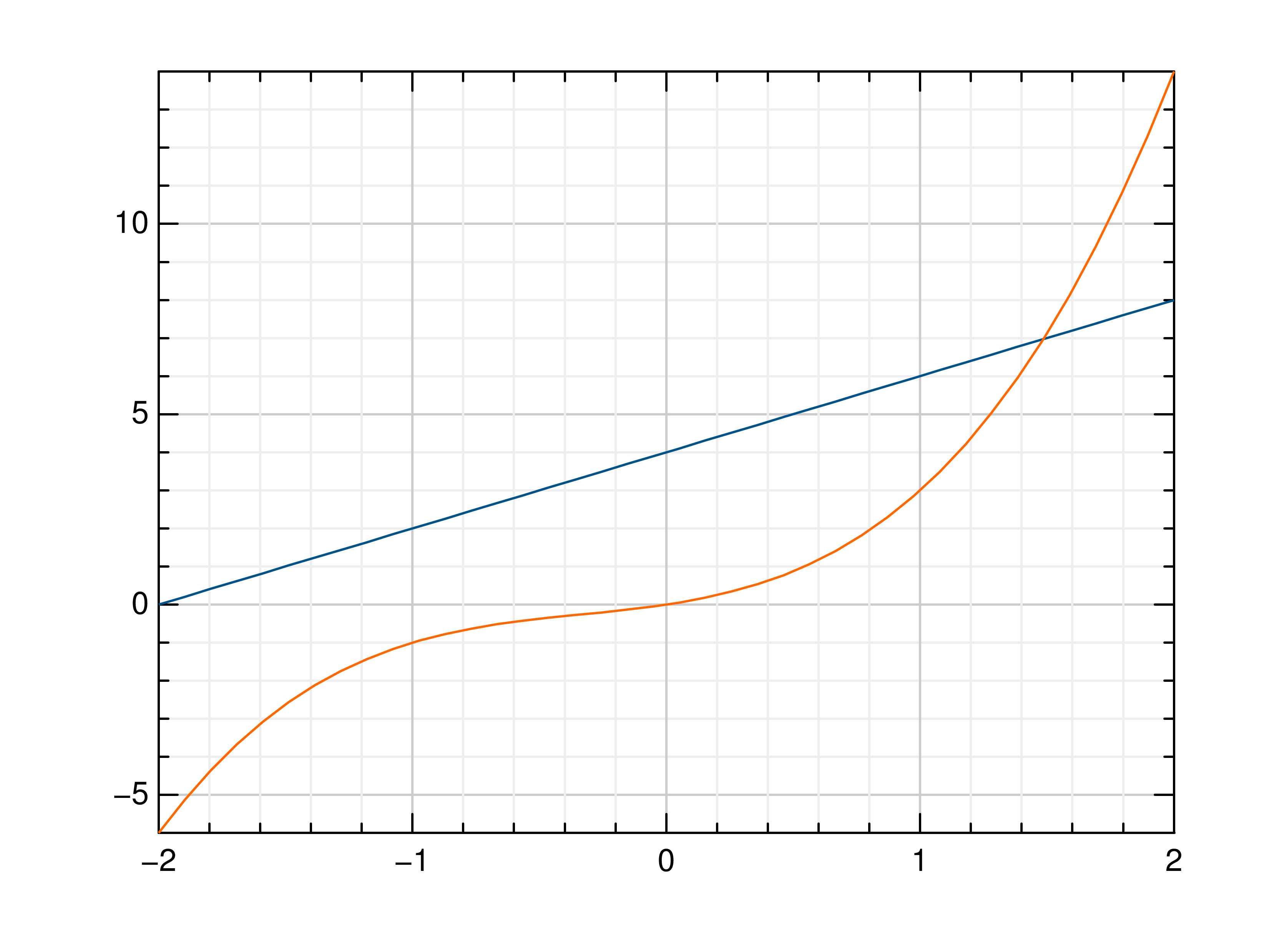gr.pygr.mlab.step(*args, **kwargs)[source]

Draw one or more step or staircase plots.

This function can receive one or more of the following:

• x values and y values, or
• x values and a callable to determine y values, or
• y values only, with their indices as x values
Parameters: args – the data to plot where – pre, mid or post, to decide where the step between two y values should be placed

Usage examples:

>>> # Create example data
>>> x = np.linspace(-2, 2, 40)
>>> y = 2*x+4
>>> # Plot x and y
>>> mlab.step(x, y)
>>> # Plot x and a callable
>>> mlab.step(x, lambda x: x**3 + x**2 + x)
>>> # Plot y, using its indices for the x values
>>> mlab.step(y)
>>> # Use next y step directly after x each position
>>> mlab.step(y, where='pre')
>>> # Use next y step between two x positions
>>> mlab.step(y, where='mid')
>>> # Use next y step immediately before next x position
>>> mlab.step(y, where='post')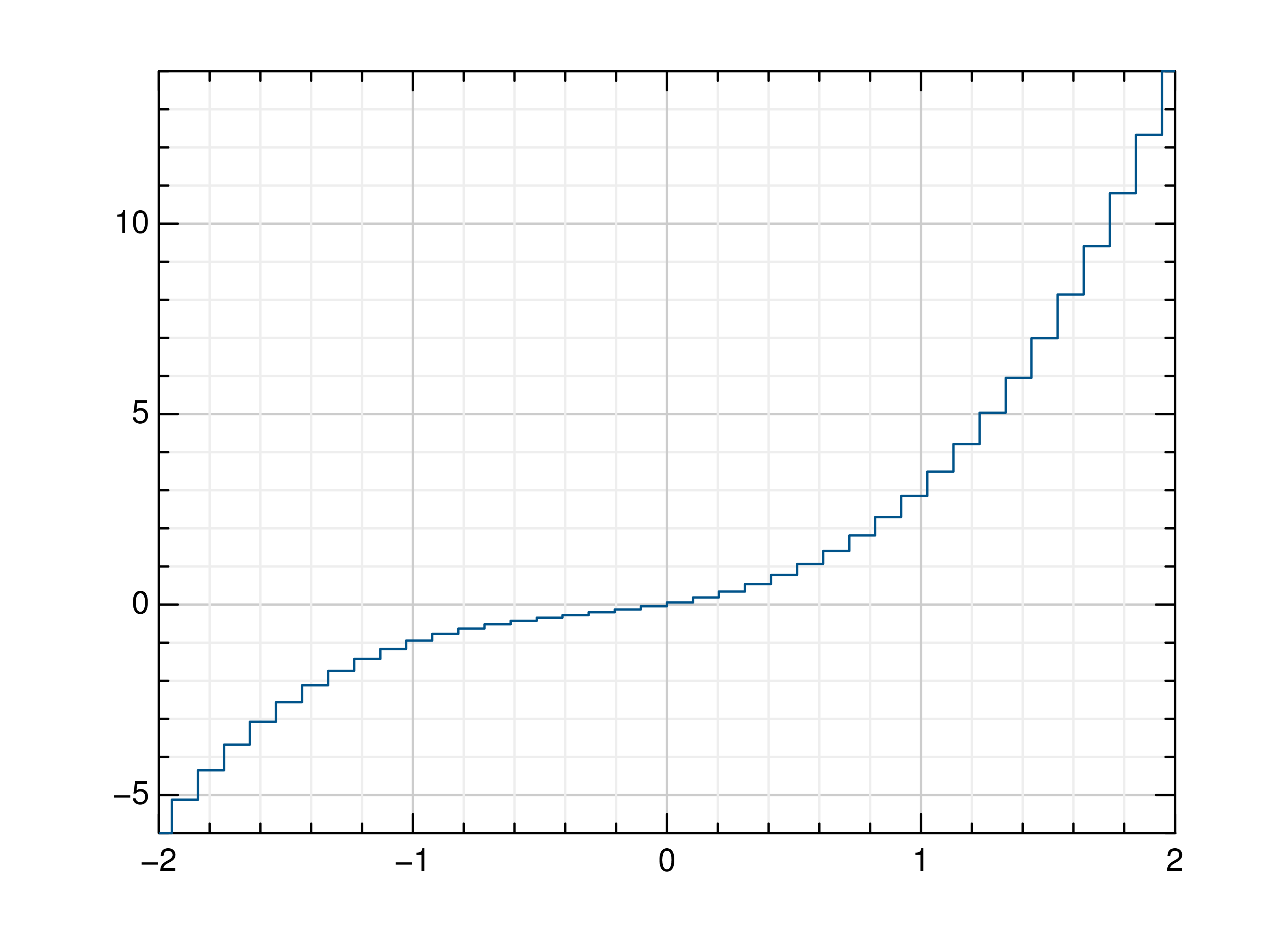gr.pygr.mlab.plot3(*args, **kwargs)[source]

Draw one or more three-dimensional line plots.

Parameters: x – the x coordinates to plot y – the y coordinates to plot z – the z coordinates to plot

Usage examples:

>>> # Create example data
>>> x = np.linspace(0, 30, 1000)
>>> y = np.cos(x) * x
>>> z = np.sin(x) * x
>>> # Plot the points
>>> mlab.plot3(x, y, z)gr.pygr.mlab.polar(*args, **kwargs)[source]

Draw one or more polar plots.

This function can receive one or more of the following:

• angle values and radius values, or
• angle values and a callable to determine radius values
Parameters: args – the data to plot

Usage examples:

>>> # Create example data
>>> angles = np.linspace(0, 2*math.pi, 40)
>>> radii = np.linspace(0, 2, 40)
>>> # Plot angles and radii
>>> # Plot angles and a callable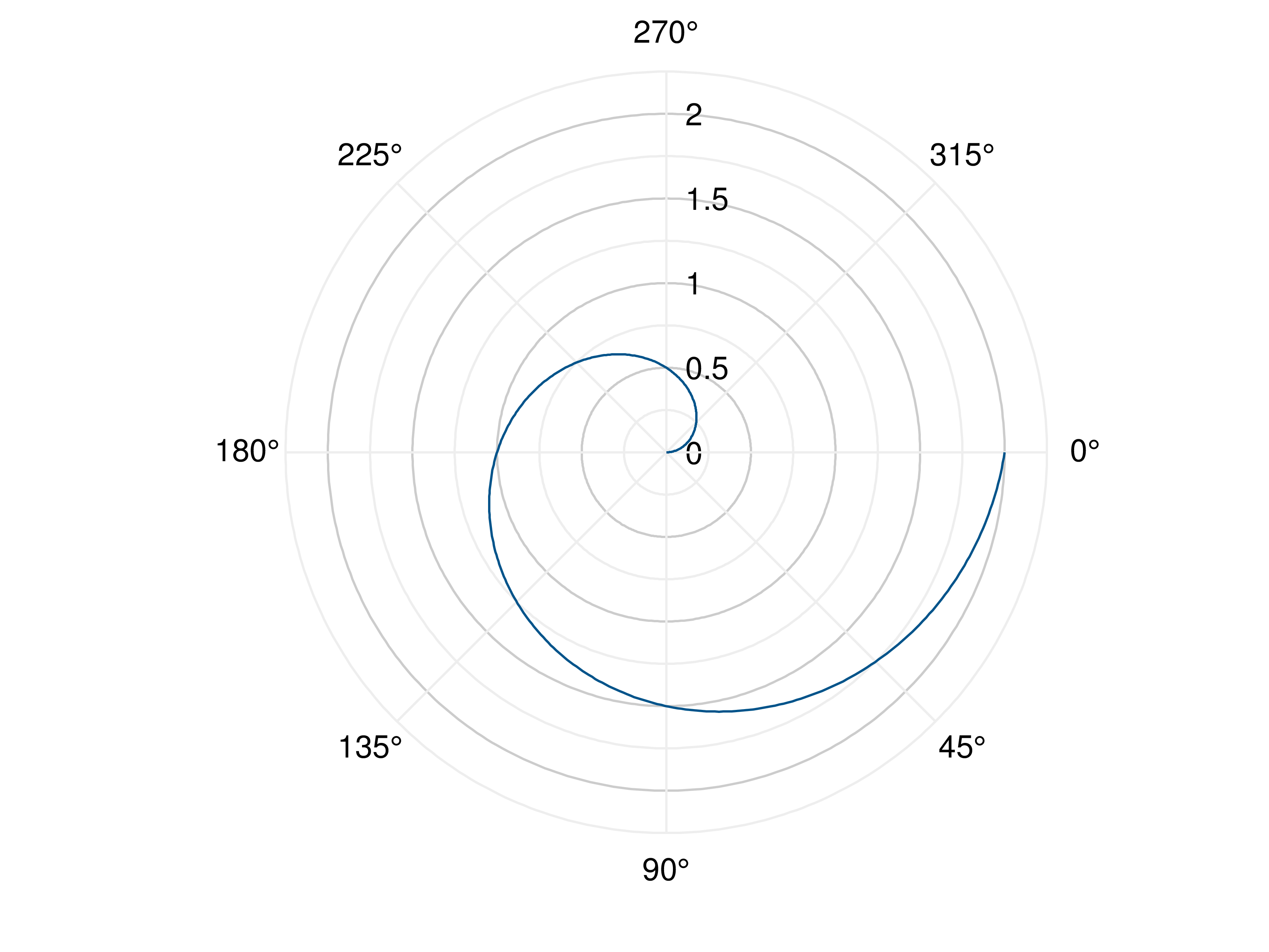### Scatter Plots¶

gr.pygr.mlab.scatter(*args, **kwargs)[source]

Draw one or more scatter plots.

This function can receive one or more of the following:

• x values and y values, or
• x values and a callable to determine y values, or
• y values only, with their indices as x values

Additional to x and y values, you can provide values for the markers’ size and color. Size values will determine the marker size in percent of the regular size, and color values will be used in combination with the current colormap.

Parameters: args – the data to plot

Usage examples:

>>> # Create example data
>>> x = np.linspace(-2, 2, 40)
>>> y = 0.2*x+0.4
>>> # Plot x and y
>>> mlab.scatter(x, y)
>>> # Plot x and a callable
>>> mlab.scatter(x, lambda x: 0.2*x + 0.4)
>>> # Plot y, using its indices for the x values
>>> mlab.scatter(y)
>>> # Plot a diagonal with increasing size and color
>>> x = np.linspace(0, 1, 11)
>>> y = np.linspace(0, 1, 11)
>>> s = np.linspace(50, 400, 11)
>>> c = np.linspace(0, 255, 11)
>>> mlab.scatter(x, y, s, c)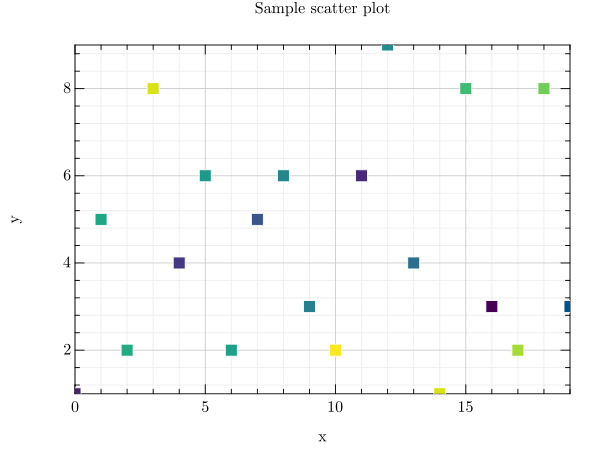gr.pygr.mlab.scatter3(x, y, z, c=None, *args, **kwargs)[source]

Draw one or more three-dimensional scatter plots.

Additional to x, y and z values, you can provide values for the markers’ color. Color values will be used in combination with the current colormap.

Parameters: x – the x coordinates to plot y – the y coordinates to plot z – the z coordinates to plot c – the optional color values to plot

Usage examples:

>>> # Create example data
>>> x = np.random.uniform(-1, 1, 100)
>>> y = np.random.uniform(-1, 1, 100)
>>> z = np.random.uniform(-1, 1, 100)
>>> c = np.random.uniform(1, 1000, 100)
>>> # Plot the points
>>> mlab.scatter3(x, y, z)
>>> # Plot the points with colors
>>> mlab.scatter3(x, y, z, c)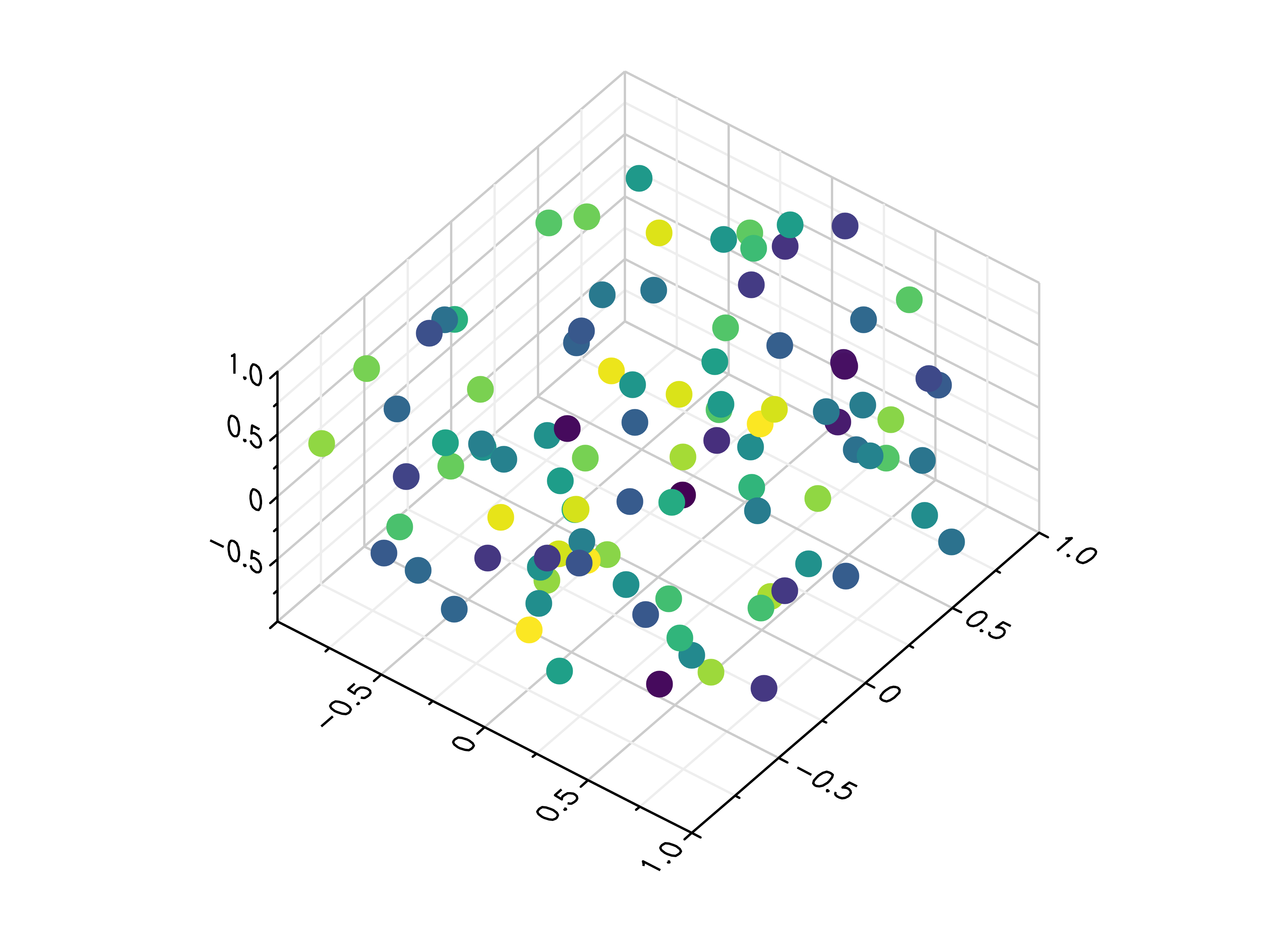### Quiver Plots¶

gr.pygr.mlab.quiver(x, y, u, v, **kwargs)[source]

Draw a quiver plot.

This function draws arrows to visualize a vector for each point of a grid.

Parameters: x – the X coordinates of the grid y – the Y coordinates of the grid u – the U component for each point on the grid v – the V component for each point on the grid

Usage examples:

>>> # Create example data
>>> x = np.linspace(-1, 1, 30)
>>> y = np.linspace(-1, 1, 20)
>>> u = np.repeat(x[np.newaxis, :], len(y), axis=0)
>>> v = np.repeat(y[:, np.newaxis], len(x), axis=1)
>>> # Draw arrows on grid
>>> mlab.quiver(x, y, u, v)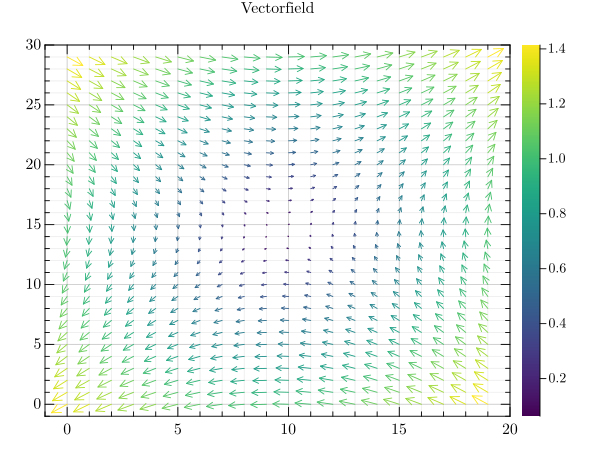### Stem Plots¶

gr.pygr.mlab.stem(*args, **kwargs)[source]

Draw a stem plot.

This function can receive one or more of the following:

• x values and y values, or
• x values and a callable to determine y values, or
• y values only, with their indices as x values
Parameters: args – the data to plot

Usage examples:

>>> # Create example data
>>> x = np.linspace(-2, 2, 40)
>>> y = 0.2*x+0.4
>>> # Plot x and y
>>> mlab.stem(x, y)
>>> # Plot x and a callable
>>> mlab.stem(x, lambda x: x**3 + x**2 + x + 6)
>>> # Plot y, using its indices for the x values
>>> mlab.stem(y)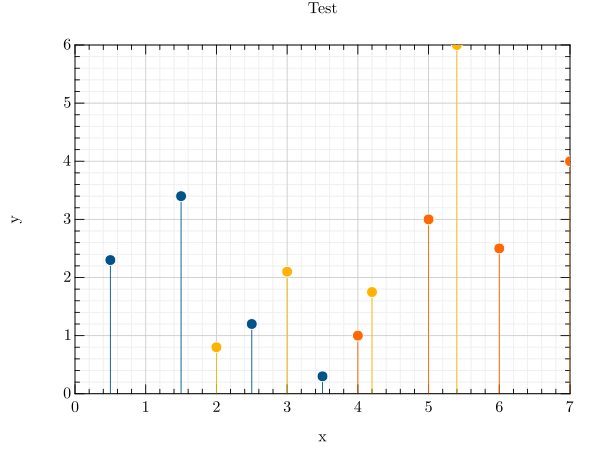### Histograms¶

gr.pygr.mlab.histogram(x, num_bins=0, **kwargs)[source]

Draw a histogram.

If num_bins is 0, this function computes the number of bins as $$\text{round}(3.3\cdot\log_{10}(n))+1$$ with n as the number of elements in x, otherwise the given number of bins is used for the histogram.

Parameters: x – the values to draw as histogram num_bins – the number of bins in the histogram

Usage examples:

>>> # Create example data
>>> x = np.random.uniform(-1, 1, 100)
>>> # Draw the histogram
>>> mlab.histogram(x)
>>> # Draw the histogram with 19 bins
>>> mlab.histogram(x, num_bins=19)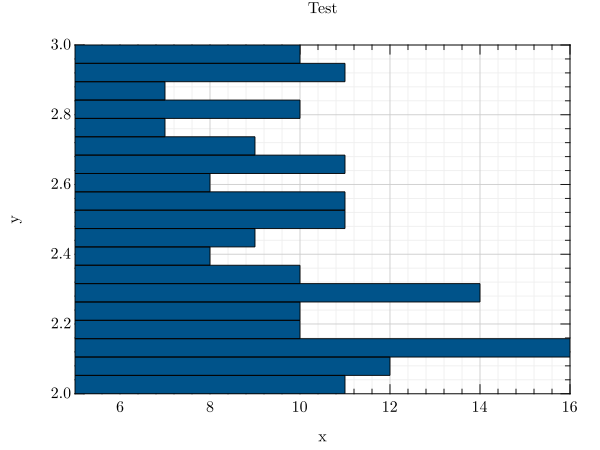gr.pygr.mlab.hexbin(*args, **kwargs)[source]

Draw a hexagon binning plot.

This function uses hexagonal binning and the the current colormap to display a series of points. It can receive one or more of the following:

• x values and y values, or
• x values and a callable to determine y values, or
• y values only, with their indices as x values
Parameters: args – the data to plot

Usage examples:

>>> # Create example data
>>> x = np.random.normal(0, 1, 100000)
>>> y = np.random.normal(0, 1, 100000)
>>> # Draw the hexbin plot
>>> mlab.hexbin(x, y)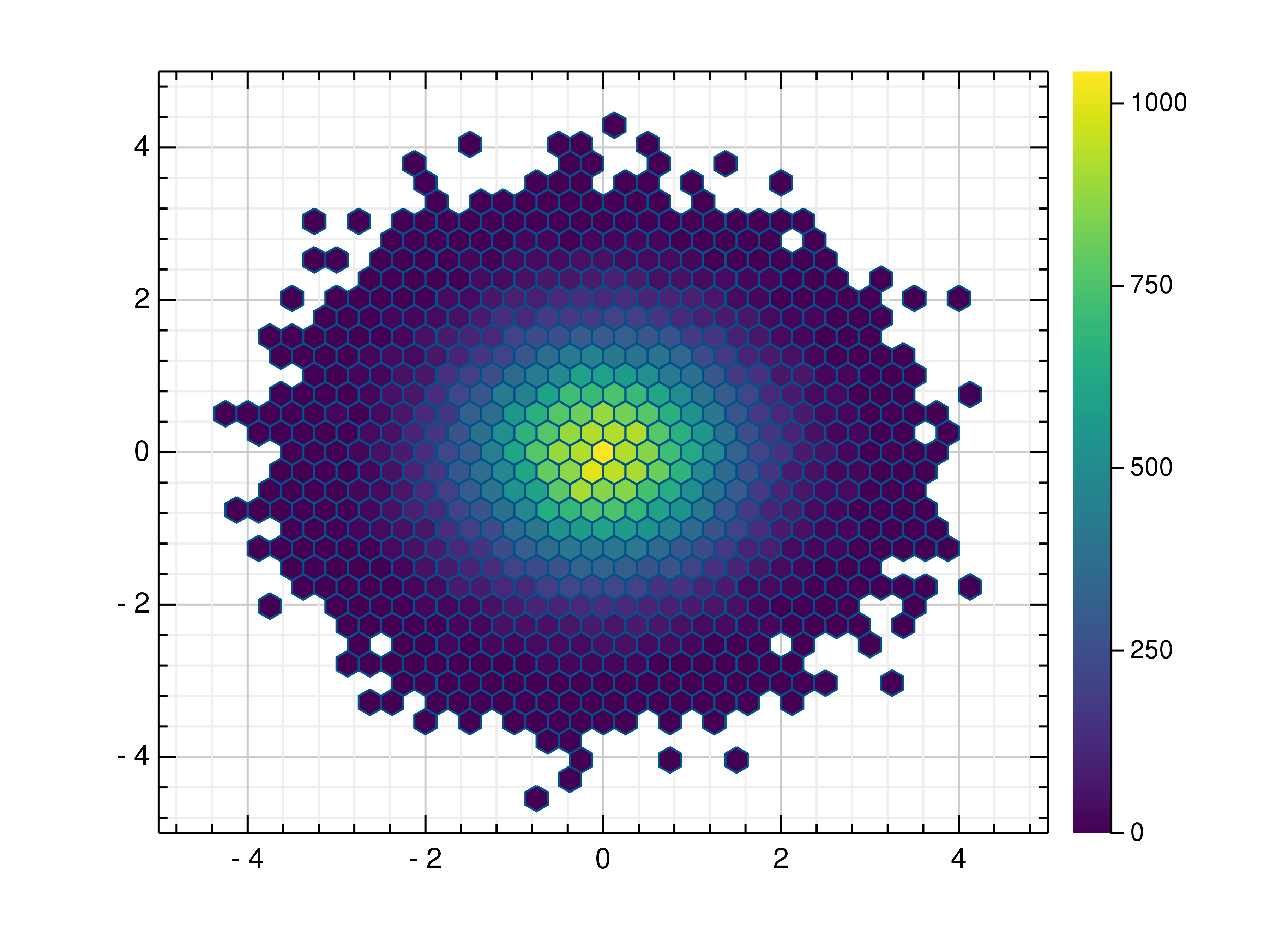### Contour Plots¶

gr.pygr.mlab.contour(*args, **kwargs)[source]

Draw a contour plot.

This function uses the current colormap to display a either a series of points or a two-dimensional array as a contour plot. It can receive one or more of the following:

• x values, y values and z values, or
• N x values, M y values and z values on a NxM grid, or
• N x values, M y values and a callable to determine z values

If a series of points is passed to this function, their values will be interpolated on a grid. For grid points outside the convex hull of the provided points, a value of 0 will be used.

Parameters: args – the data to plot levels – Number of contour lines

Usage examples:

>>> # Create example point data
>>> x = np.random.uniform(-4, 4, 100)
>>> y = np.random.uniform(-4, 4, 100)
>>> z = np.sin(x) + np.cos(y)
>>> # Draw the contour plot
>>> mlab.contour(x, y, z)
>>> # Create example grid data
>>> x = np.linspace(-2, 2, 40)
>>> y = np.linspace(0, np.pi, 20)
>>> z = np.sin(x[:, np.newaxis]) + np.cos(y[np.newaxis, :])
>>> # Draw the contour plot
>>> mlab.contour(x, y, z, levels=10)
>>> # Draw the contour plot using a callable
>>> mlab.contour(x, y, lambda x, y: np.sin(x) + np.cos(y))gr.pygr.mlab.contourf(*args, **kwargs)[source]

Draw a filled contour plot.

This function uses the current colormap to display a either a series of points or a two-dimensional array as a filled contour plot. It can receive one or more of the following:

• x values, y values and z values, or
• N x values, M y values and z values on a NxM grid, or
• N x values, M y values and a callable to determine z values

If a series of points is passed to this function, their values will be interpolated on a grid. For grid points outside the convex hull of the provided points, a value of 0 will be used.

Parameters: args – the data to plot levels – Number of contour lines

Usage examples:

>>> # Create example point data
>>> x = np.random.uniform(-4, 4, 100)
>>> y = np.random.uniform(-4, 4, 100)
>>> z = np.sin(x) + np.cos(y)
>>> # Draw the filled contour plot
>>> mlab.contourf(x, y, z)
>>> # Create example grid data
>>> x = np.linspace(-2, 2, 40)
>>> y = np.linspace(0, np.pi, 20)
>>> z = np.sin(x[:, np.newaxis]) + np.cos(y[np.newaxis, :])
>>> # Draw the filled contour plot
>>> mlab.contourf(x, y, z, levels=10)
>>> # Draw the filled contour plot using a callable
>>> mlab.contourf(x, y, lambda x, y: np.sin(x) + np.cos(y))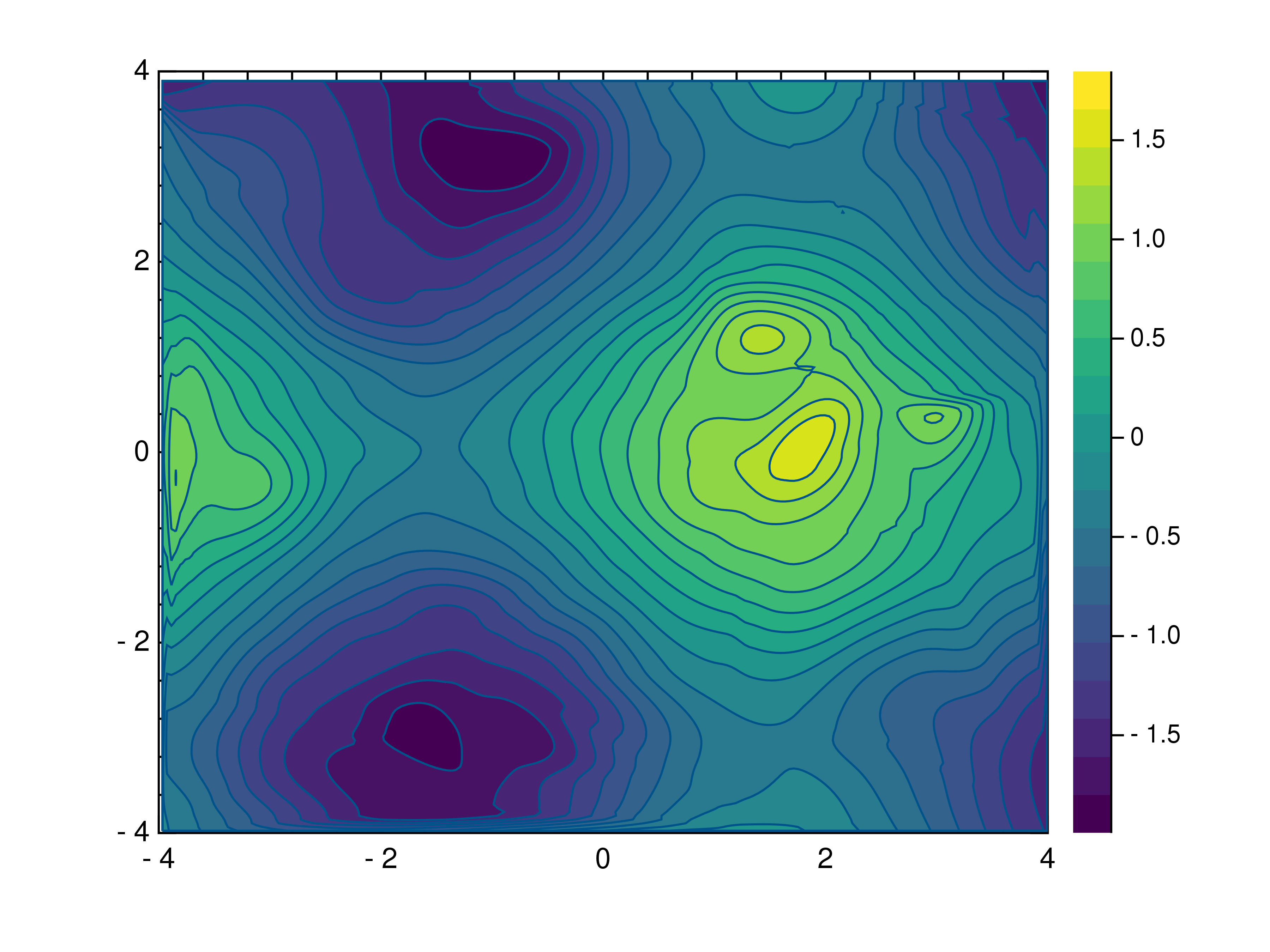gr.pygr.mlab.tricont(x, y, z, *args, **kwargs)[source]

Draw a triangular contour plot.

This function uses the current colormap to display a series of points as a triangular contour plot. It will use a Delaunay triangulation to interpolate the z values between x and y values. If the series of points is concave, this can lead to interpolation artifacts on the edges of the plot, as the interpolation may occur in very acute triangles.

Parameters: x – the x coordinates to plot y – the y coordinates to plot z – the z coordinates to plot

Usage examples:

>>> # Create example point data
>>> x = np.random.uniform(-4, 4, 100)
>>> y = np.random.uniform(-4, 4, 100)
>>> z = np.sin(x) + np.cos(y)
>>> # Draw the triangular contour plot
>>> mlab.tricont(x, y, z)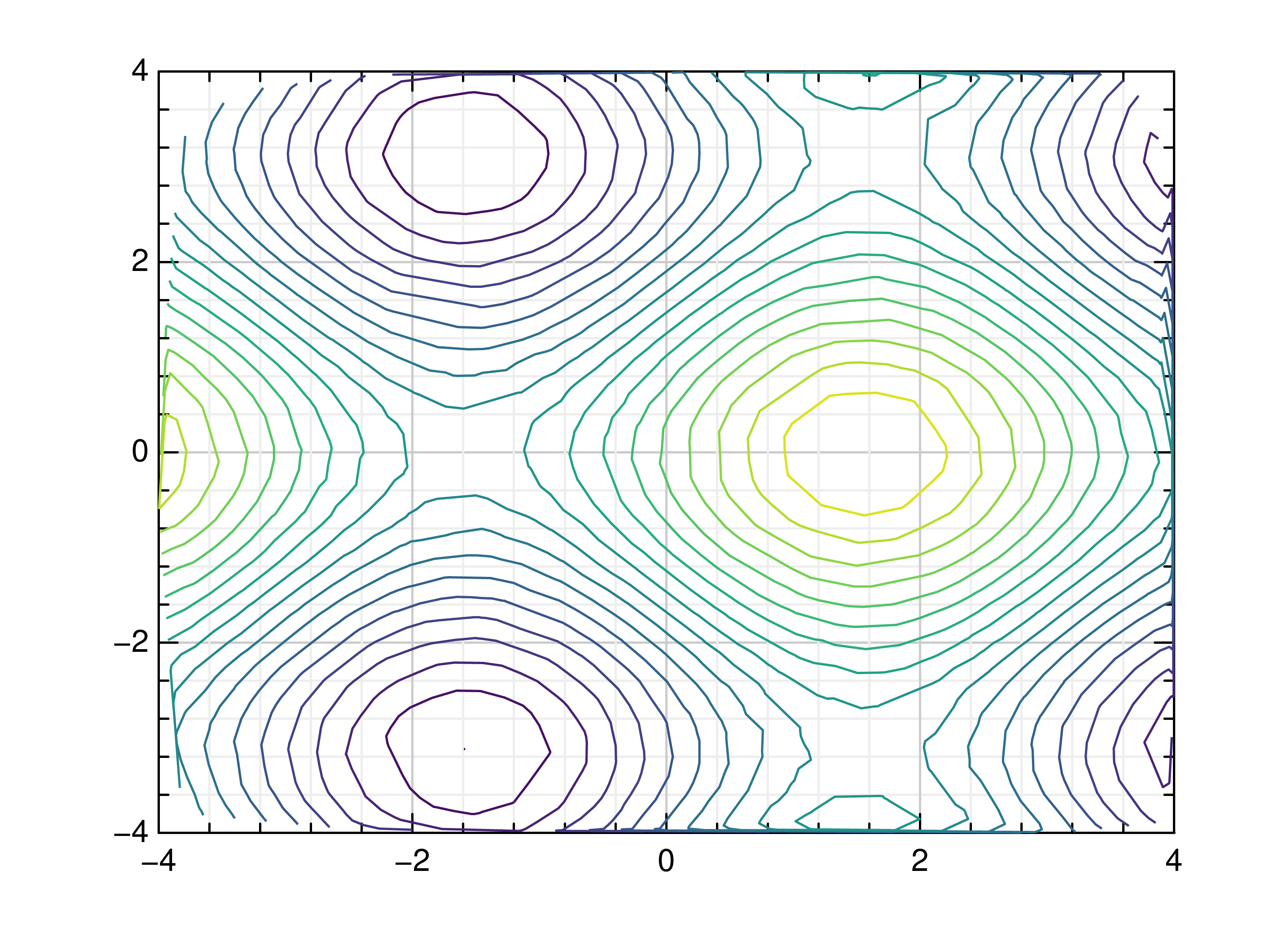### Surface Plots¶

gr.pygr.mlab.surface(*args, **kwargs)[source]

Draw a three-dimensional surface plot.

This function uses the current colormap to display a either a series of points or a two-dimensional array as a surface plot. It can receive one or more of the following:

• x values, y values and z values, or
• N x values, M y values and z values on a NxM grid, or
• N x values, M y values and a callable to determine z values

If a series of points is passed to this function, their values will be interpolated on a grid. For grid points outside the convex hull of the provided points, a value of 0 will be used.

Parameters: args – the data to plot

Usage examples:

>>> # Create example point data
>>> x = np.random.uniform(-4, 4, 100)
>>> y = np.random.uniform(-4, 4, 100)
>>> z = np.sin(x) + np.cos(y)
>>> # Draw the surface plot
>>> mlab.surface(x, y, z)
>>> # Create example grid data
>>> x = np.linspace(-2, 2, 40)
>>> y = np.linspace(0, np.pi, 20)
>>> z = np.sin(x[:, np.newaxis]) + np.cos(y[np.newaxis, :])
>>> # Draw the surface plot
>>> mlab.surface(x, y, z)
>>> # Draw the surface plot using a callable
>>> mlab.surface(x, y, lambda x, y: np.sin(x) + np.cos(y))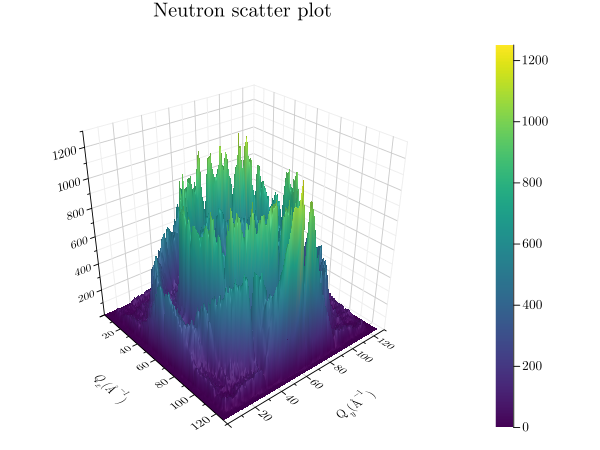gr.pygr.mlab.trisurf(*args, **kwargs)[source]

Draw a triangular surface plot.

This function uses the current colormap to display a series of points as a triangular surface plot. It will use a Delaunay triangulation to interpolate the z values between x and y values. If the series of points is concave, this can lead to interpolation artifacts on the edges of the plot, as the interpolation may occur in very acute triangles.

Parameters: x – the x coordinates to plot y – the y coordinates to plot z – the z coordinates to plot

Usage examples:

>>> # Create example point data
>>> x = np.random.uniform(-4, 4, 100)
>>> y = np.random.uniform(-4, 4, 100)
>>> z = np.sin(x) + np.cos(y)
>>> # Draw the triangular surface plot
>>> mlab.trisurf(x, y, z)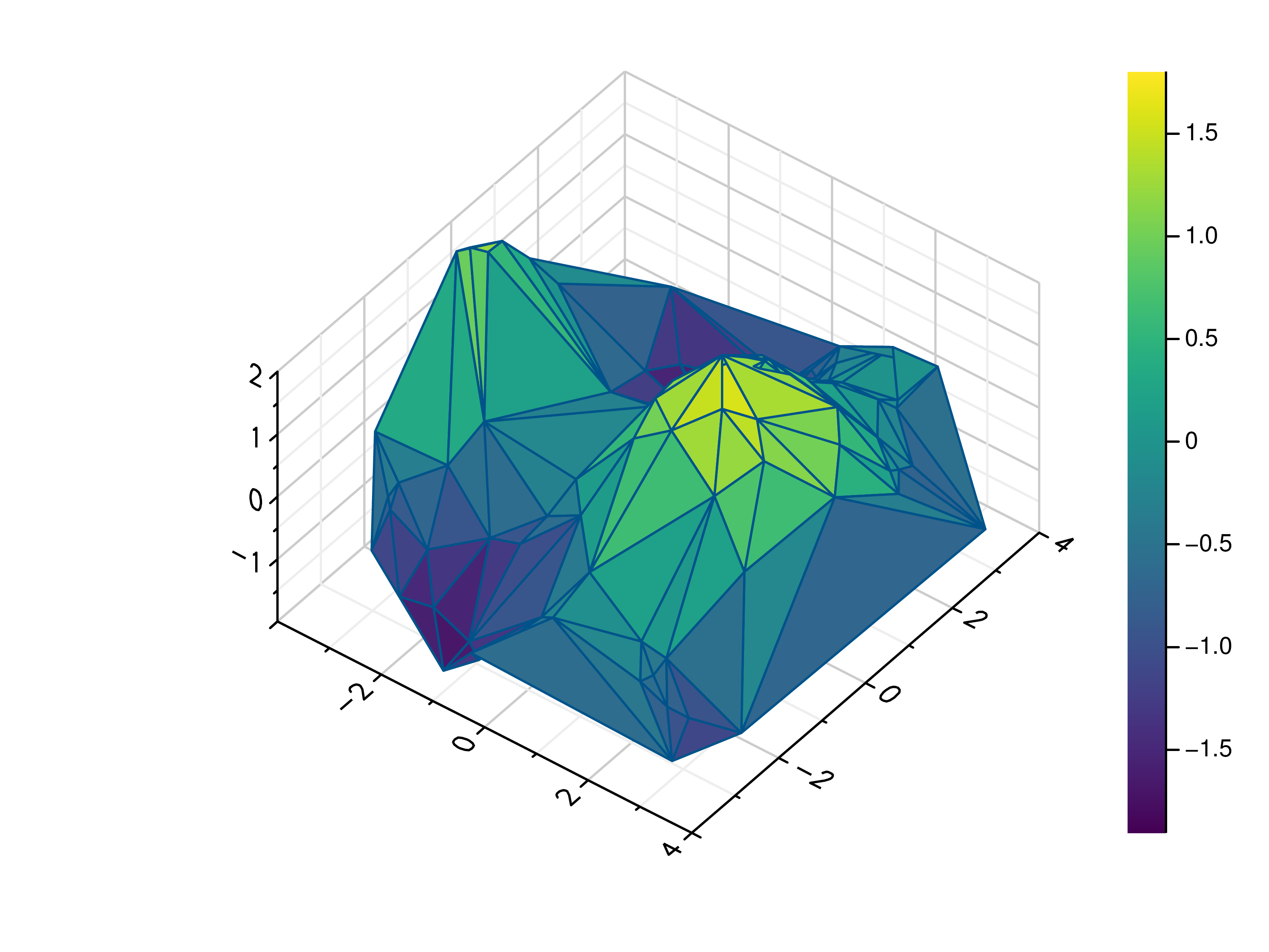gr.pygr.mlab.wireframe(*args, **kwargs)[source]

Draw a three-dimensional wireframe plot.

This function uses the current colormap to display a either a series of points or a two-dimensional array as a wireframe plot. It can receive one or more of the following:

• x values, y values and z values, or
• N x values, M y values and z values on a NxM grid, or
• N x values, M y values and a callable to determine z values

If a series of points is passed to this function, their values will be interpolated on a grid. For grid points outside the convex hull of the provided points, a value of 0 will be used.

Parameters: args – the data to plot

Usage examples:

>>> # Create example point data
>>> x = np.random.uniform(-4, 4, 100)
>>> y = np.random.uniform(-4, 4, 100)
>>> z = np.sin(x) + np.cos(y)
>>> # Draw the wireframe plot
>>> mlab.wireframe(x, y, z)
>>> # Create example grid data
>>> x = np.linspace(-2, 2, 40)
>>> y = np.linspace(0, np.pi, 20)
>>> z = np.sin(x[:, np.newaxis]) + np.cos(y[np.newaxis, :])
>>> # Draw the wireframe plot
>>> mlab.wireframe(x, y, z)
>>> # Draw the wireframe plot using a callable
>>> mlab.wireframe(x, y, lambda x, y: np.sin(x) + np.cos(y))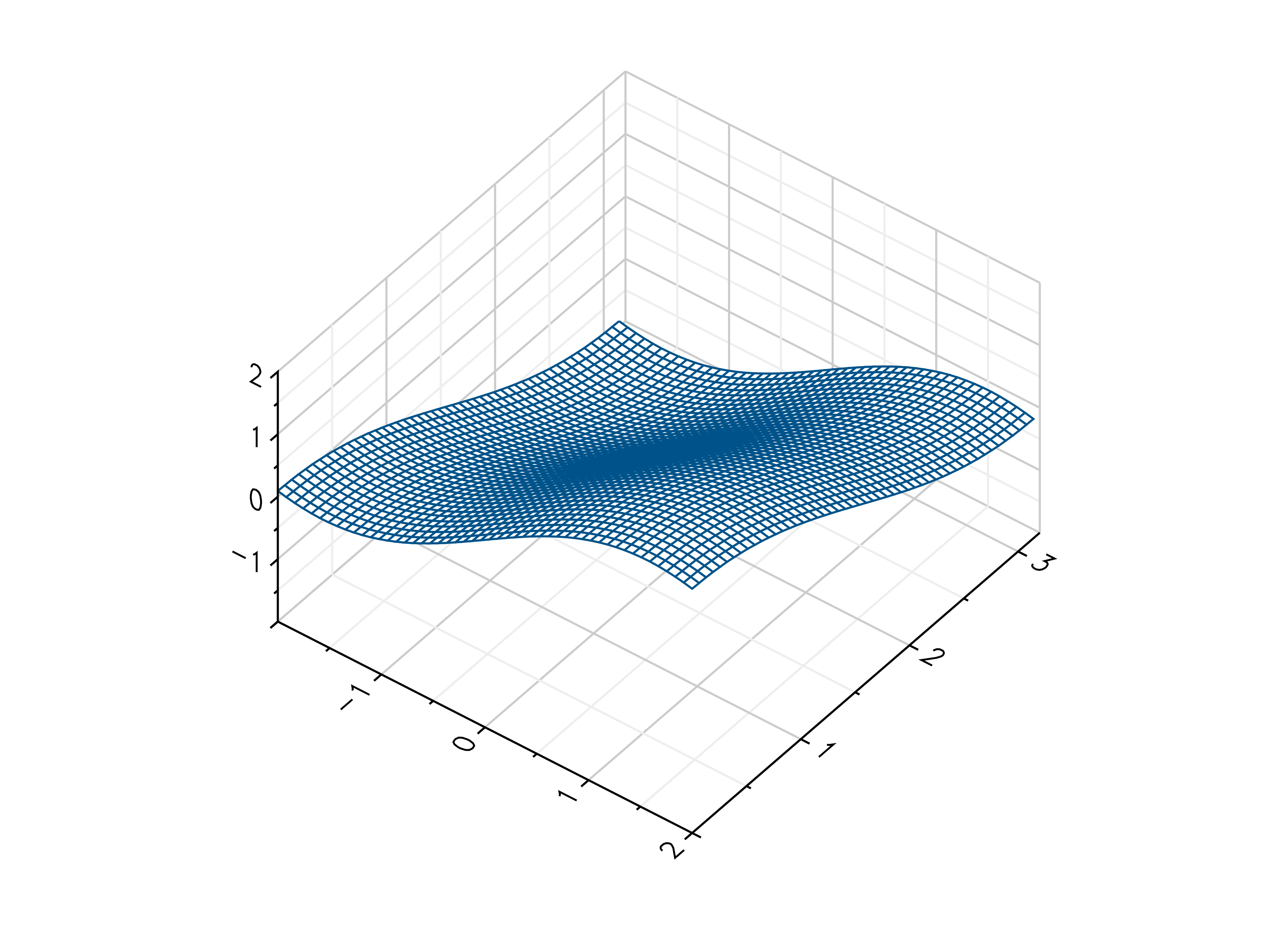### Heatmaps¶

gr.pygr.mlab.heatmap(data, **kwargs)[source]

Draw a heatmap.

This function uses the current colormap to display a two-dimensional array as a heatmap. The array is drawn with its first value in the upper left corner, so in some cases it may be neccessary to flip the columns (see the example below).

By default the function will use the row and column indices for the x- and y-axes, so setting the axis limits is recommended. Also note that the values in the array must lie within the current z-axis limits so it may be neccessary to adjust these limits or clip the range of array values.

Parameters: data – the heatmap data

Usage examples:

>>> # Create example data
>>> x = np.linspace(-2, 2, 40)
>>> y = np.linspace(0, np.pi, 20)
>>> z = np.sin(x[np.newaxis, :]) + np.cos(y[:, np.newaxis])
>>> # Draw the heatmap
>>> mlab.heatmap(z[::-1, :], xlim=(-2, 2), ylim=(0, np.pi))gr.pygr.mlab.shade(*args, **kwargs)[source]

Draw a point or line based heatmap.

This function uses the current colormap to display a series of points or polylines. For line data, NaN values can be used as separator.

Parameters: args – the data to plot xform – the transformation type used for color mapping

The available transformation types are:

 XFORM_BOOLEAN 0 boolean XFORM_LINEAR 1 linear XFORM_LOG 2 logarithmic XFORM_LOGLOG 3 double logarithmic XFORM_CUBIC 4 cubic XFORM_EQUALIZED 5 histogram equalized

Usage examples:

>>> # Create point data
>>> x = np.random.normal(size=100000)
>>> y = np.random.normal(size=100000)
>>> # Create line data with NaN as polyline separator
>>> x = np.concatenate((np.random.normal(size=10000), [np.nan], np.random.normal(loc=5, size=10000)))
>>> y = np.concatenate((np.random.normal(size=10000), [np.nan], np.random.normal(loc=5, size=10000)))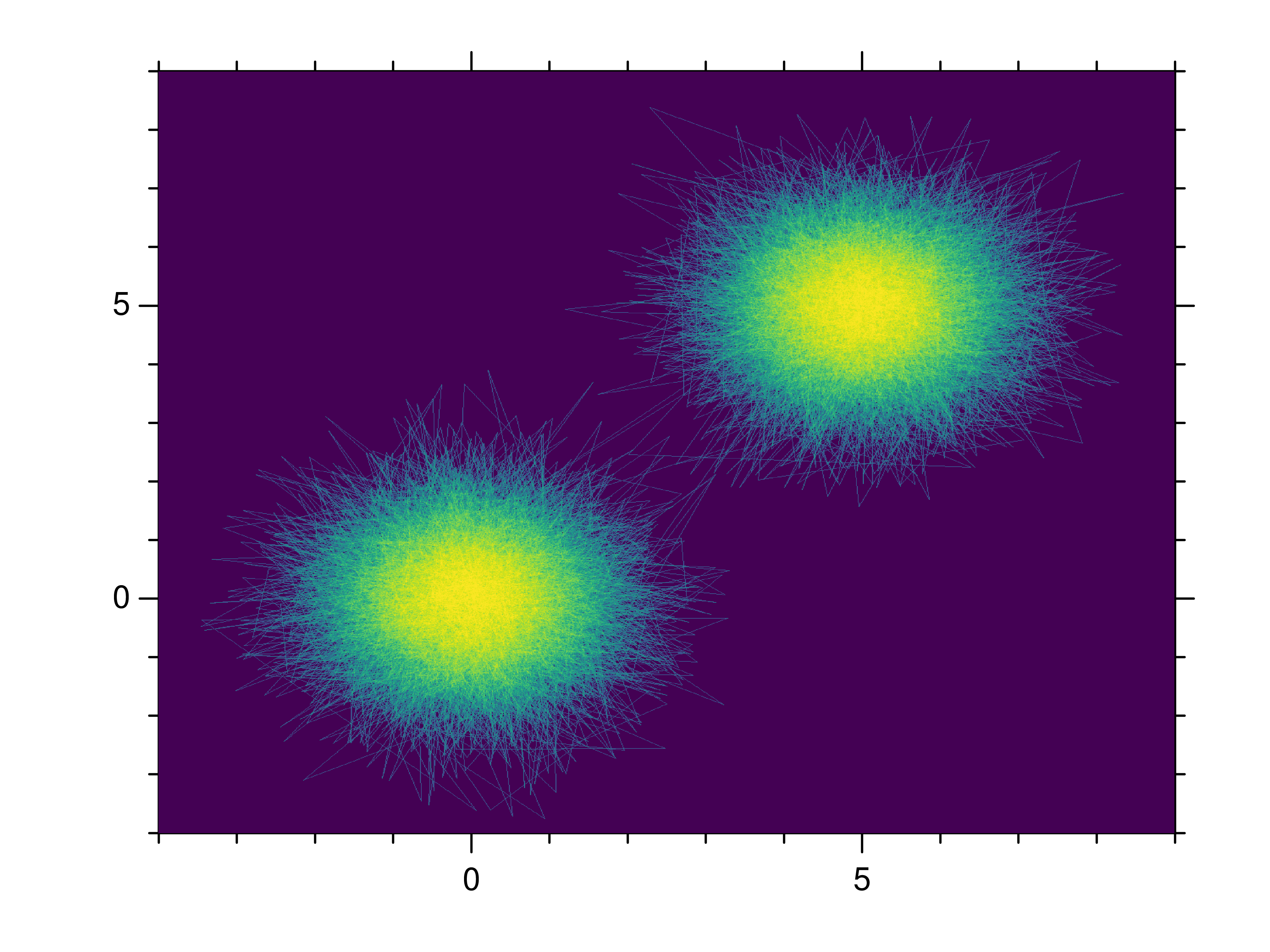### Images¶

gr.pygr.mlab.imshow(image, **kwargs)[source]

Draw an image.

This function can draw an image either from reading a file or using a two-dimensional array and the current colormap.

Parameters: image – an image file name or two-dimensional array

Usage examples:

>>> # Create example data
>>> x = np.linspace(-2, 2, 40)
>>> y = np.linspace(0, np.pi, 20)
>>> z = np.sin(x[np.newaxis, :]) + np.cos(y[:, np.newaxis])
>>> # Draw an image from a 2d array
>>> mlab.imshow(z)
>>> # Draw an image from a file
>>> mlab.imshow("example.png")### Isosurfaces¶

gr.pygr.mlab.isosurface(v, **kwargs)[source]

Draw an isosurface.

This function can draw an isosurface from a three-dimensional numpy array. Values greater than the isovalue will be seen as outside the isosurface, while values less than the isovalue will be seen as inside the isosurface.

Parameters: v – the volume data isovalue – the isovalue

Usage examples:

>>> # Create example data
>>> x = np.linspace(-1, 1, 40)[:, np.newaxis, np.newaxis]
>>> y = np.linspace(-1, 1, 40)[np.newaxis, :, np.newaxis]
>>> z = np.linspace(-1, 1, 40)[np.newaxis, np.newaxis, :]
>>> v = 1-(x**2 + y**2 + z**2)**0.5
>>> # Draw the isosurace.
>>> mlab.isosurface(v, isovalue=0.2)### Volume Rendering¶

gr.pygr.mlab.volume(v, **kwargs)[source]

Draw a volume.

This function can draw a three-dimensional numpy array using volume rendering. The volume data is reduced to a two-dimensional image using an emission or absorption model or by a maximum intensity projection. After the projection the current colormap is applied to the result.

Parameters: v – the volume data algorithm – the algorithm used to reduce the volume data. Available algorithms are “maximum”, “emission” and “absorption”. dmin – the minimum data value when applying the colormap dmax – the maximum data value when applying the colormap

Usage examples:

>>> # Create example data
>>> x = np.linspace(-1, 1, 40)[:, np.newaxis, np.newaxis]
>>> y = np.linspace(-1, 1, 40)[np.newaxis, :, np.newaxis]
>>> z = np.linspace(-1, 1, 40)[np.newaxis, np.newaxis, :]
>>> v = 1 - (x**2 + y**2 + z**2)**0.5 - np.random.uniform(0, 0.25, (40, 40, 40))
>>> # Draw the 3d volume data
>>> mlab.volume(v)
>>> # Draw the 3d volume data using an emission model
>>> mlab.volume(v, algorithm='emission', dmin=0.1, dmax=0.4)## Attribute Functions¶

gr.pygr.mlab.title(title='')[source]

Set the plot title.

The plot title is drawn using the extended text function gr.textext(). You can use a subset of LaTeX math syntax, but will need to escape certain characters, e.g. parentheses. For more information see the documentation of gr.textext().

Parameters: title – the plot title

Usage examples:

>>> # Set the plot title to "Example Plot"
>>> mlab.title("Example Plot")
>>> # Clear the plot title
>>> mlab.title()

gr.pygr.mlab.legend(*labels, **kwargs)[source]

Set the labels and location for the legend of the current plot.

The labels for the legend are drawn using the extended text function gr.textext(). You can use a subset of LaTeX math syntax, but will need to escape certain characters, e.g. parentheses. For more information see the documentation of gr.textext().

Parameters: labels – the labels for each graph in the current plot location – the location of the legend (from 1 to 10)

Usage examples:

>>> # Set the labels for the two graphs "f(x)" and "g(x)"
>>> mlab.legend("f$$x$$", "g$$x$$")
>>> # Set the labels and draws the legend in the lower right corner
>>> mlab.legend("f$$x$$", "g$$x$$", location=4)
>>> # Resets the legend
>>> mlab.legend()

gr.pygr.mlab.xlabel(x_label='')[source]

Set the x-axis label.

The axis labels are drawn using the extended text function gr.textext(). You can use a subset of LaTeX math syntax, but will need to escape certain characters, e.g. parentheses. For more information see the documentation of gr.textext().

Parameters: x_label – the x-axis label

Usage examples:

>>> # Set the x-axis label to "x"
>>> mlab.xlabel("x")
>>> # Clear the x-axis label
>>> mlab.xlabel()

gr.pygr.mlab.ylabel(y_label='')[source]

Set the y-axis label.

The axis labels are drawn using the extended text function gr.textext(). You can use a subset of LaTeX math syntax, but will need to escape certain characters, e.g. parentheses. For more information see the documentation of gr.textext().

Parameters: y_label – the y-axis label

Usage examples:

>>> # Set the y-axis label to "y$$x$$"
>>> mlab.ylabel("y$$x$$")
>>> # Clear the y-axis label
>>> mlab.ylabel()

gr.pygr.mlab.zlabel(z_label='')[source]

Set the z-axis label.

The axis labels are drawn using the extended text function gr.textext(). You can use a subset of LaTeX math syntax, but will need to escape certain characters, e.g. parentheses. For more information see the documentation of gr.textext().

Parameters: z_label – the z-axis label

Usage examples:

>>> # Set the z-axis label to "z(x, y)"
>>> mlab.zlabel("z$$x, y$$")
>>> # Clear the z-axis label
>>> mlab.zlabel()

gr.pygr.mlab.dlabel(d_label='')[source]

Set the volume intensity label.

This label is drawn using the extended text function gr.textext(). You can use a subset of LaTeX math syntax, but will need to escape certain characters, e.g. parentheses. For more information see the documentation of gr.textext().

Parameters: d_label – the volume intensity label

Usage examples:

>>> # Set the volume intensity label to "Intensity"
>>> mlab.dlabel("Intensity")
>>> # Clear the volume intensity label
>>> mlab.dlabel()

gr.pygr.mlab.xlim(x_min=None, x_max=None, adjust=True)[source]

Set the limits for the x-axis.

The x-axis limits can either be passed as individual arguments or as a tuple of (x_min, x_max). Setting either limit to None will cause it to be automatically determined based on the data, which is the default behavior.

Parameters: x_min – the x-axis lower limit, or None to use an automatic lower limit, or a tuple of both x-axis limits x_max – the x-axis upper limit, or None to use an automatic upper limit, or None if both x-axis limits were passed as first argument adjust – whether or not the limits may be adjusted

Usage examples:

>>> # Set the x-axis limits to -1 and 1
>>> mlab.xlim(-1, 1)
>>> # Set the x-axis limits to -1 and 1 using a tuple
>>> mlab.xlim((-1, 1))
>>> # Reset the x-axis limits to be determined automatically
>>> mlab.xlim()
>>> # Reset the x-axis upper limit and set the lower limit to 0
>>> mlab.xlim(0, None)
>>> # Reset the x-axis lower limit and set the upper limit to 1
>>> mlab.xlim(None, 1)

gr.pygr.mlab.ylim(y_min=None, y_max=None, adjust=True)[source]

Set the limits for the y-axis.

The y-axis limits can either be passed as individual arguments or as a tuple of (y_min, y_max). Setting either limit to None will cause it to be automatically determined based on the data, which is the default behavior.

Parameters: y_min – the y-axis lower limit, or None to use an automatic lower limit, or a tuple of both y-axis limits y_max – the y-axis upper limit, or None to use an automatic upper limit, or None if both y-axis limits were passed as first argument adjust – whether or not the limits may be adjusted

Usage examples:

>>> # Set the y-axis limits to -1 and 1
>>> mlab.ylim(-1, 1)
>>> # Set the y-axis limits to -1 and 1 using a tuple
>>> mlab.ylim((-1, 1))
>>> # Reset the y-axis limits to be determined automatically
>>> mlab.ylim()
>>> # Reset the y-axis upper limit and set the lower limit to 0
>>> mlab.ylim(0, None)
>>> # Reset the y-axis lower limit and set the upper limit to 1
>>> mlab.ylim(None, 1)

gr.pygr.mlab.zlim(z_min=None, z_max=None, adjust=True)[source]

Set the limits for the z-axis.

The z-axis limits can either be passed as individual arguments or as a tuple of (z_min, z_max). Setting either limit to None will cause it to be automatically determined based on the data, which is the default behavior.

Parameters: z_min – the z-axis lower limit, or None to use an automatic lower limit, or a tuple of both z-axis limits z_max – the z-axis upper limit, or None to use an automatic upper limit, or None if both z-axis limits were passed as first argument adjust – whether or not the limits may be adjusted

Usage examples:

>>> # Set the z-axis limits to -1 and 1
>>> mlab.zlim(-1, 1)
>>> # Set the z-axis limits to -1 and 1 using a tuple
>>> mlab.zlim((-1, 1))
>>> # Reset the z-axis limits to be determined automatically
>>> mlab.zlim()
>>> # Reset the z-axis upper limit and set the lower limit to 0
>>> mlab.zlim(0, None)
>>> # Reset the z-axis lower limit and set the upper limit to 1
>>> mlab.zlim(None, 1)

gr.pygr.mlab.xlog(xlog=True)[source]

Enable or disable a logarithmic scale for the x-axis.

Parameters: xlog – whether or not the x-axis should be logarithmic

Usage examples:

>>> # Enable a logarithic x-axis
>>> mlab.xlog()
>>> # Disable it again
>>> mlab.xlog(False)

gr.pygr.mlab.ylog(ylog=True)[source]

Enable or disable a logarithmic scale for the y-axis.

Parameters: ylog – whether or not the y-axis should be logarithmic

Usage examples:

>>> # Enable a logarithic y-axis
>>> mlab.ylog()
>>> # Disable it again
>>> mlab.ylog(False)

gr.pygr.mlab.zlog(zlog=True)[source]

Enable or disable a logarithmic scale for the z-axis.

Parameters: zlog – whether or not the z-axis should be logarithmic

Usage examples:

>>> # Enable a logarithic z-axis
>>> mlab.zlog()
>>> # Disable it again
>>> mlab.zlog(False)

gr.pygr.mlab.xflip(xflip=True)[source]

Enable or disable x-axis flipping/reversal.

Parameters: xflip – whether or not the x-axis should be flipped

Usage examples:

>>> # Flips/Reverses the x-axis
>>> mlab.xlog()
>>> # Restores the x-axis
>>> mlab.xlog(False)

gr.pygr.mlab.yflip(yflip=True)[source]

Enable or disable y-axis flipping/reversal.

Parameters: yflip – whether or not the y-axis should be flipped

Usage examples:

>>> # Flips/Reverses the y-axis
>>> mlab.ylog()
>>> # Restores the y-axis
>>> mlab.ylog(False)

gr.pygr.mlab.zflip(zflip=True)[source]

Enable or disable z-axis flipping/reversal.

Parameters: zflip – whether or not the z-axis should be flipped

Usage examples:

>>> # Flips/Reverses the z-axis
>>> mlab.zlog()
>>> # Restores the z-axis
>>> mlab.zlog(False)

gr.pygr.mlab.rotation(rotation)[source]

Set the 3d axis rotation of the current plot.

The rotation can be any value between 0 and 90, and controls the angle between the viewer projected onto the X-Y-plane and the x-axis.

Parameters: rotation – the 3d axis rotation

Usage examples:

>>> # Create example data
>>> x = np.random.uniform(0, 1, 100)
>>> y = np.random.uniform(0, 1, 100)
>>> z = np.random.uniform(0, 1, 100)
>>> # Set the rotation and draw an example plot
>>> mlab.rotation(45)
>>> mlab.plot3(x, y, z)

gr.pygr.mlab.tilt(tilt)[source]

Set the 3d axis tilt of the current plot.

The tilt can be any value between 0 and 90, and controls the angle between the viewer and the X-Y-plane.

Parameters: tilt – the 3d axis tilt

Usage examples:

>>> # Create example data
>>> x = np.random.uniform(0, 1, 100)
>>> y = np.random.uniform(0, 1, 100)
>>> z = np.random.uniform(0, 1, 100)
>>> # Set the tilt and draw an example plot
>>> mlab.tilt(45)
>>> mlab.plot3(x, y, z)

gr.pygr.mlab.colormap(colormap='')[source]

Get or set the colormap for the current plot or enable manual colormap control.

Parameters: colormap – The name of a gr colormap One of the gr colormap constants (gr.COLORMAP_…) A list of red-green-blue tuples as colormap A dict mapping a normalized position to the corresponding red-green-blue tuple None, if the colormap should use the current colors set by gr.setcolorrep() No parameter or an empty string (default) to get the colormap as a list of red-green-blue tuples

Usage examples:

>>> # Use one of the built-in colormap names
>>> mlab.colormap('viridis')
>>> # Use one of the built-in colormap constants
>>> mlab.colormap(gr.COLORMAP_BWR)
>>> # Use a list of red-green-blue tuples as colormap
>>> mlab.colormap([(0, 0, 1), (1, 1, 1), (1, 0, 0)])
>>> # Use a dict mapping a normalized position to the corresponding red-green-blue tuple as colormap
>>> mlab.colormap({0.0: (0, 0, 1), 0.25: (1, 1, 1), 1.0: (1, 0, 0)})
>>> # Use a custom colormap using gr.setcolorrep directly
>>> for i in range(256):
...     gr.setcolorrep(1.0-i/255.0, 1.0, i/255.0)
...
>>> mlab.colormap(None)
>>> # Get the current colormap as list of red-green-blue tuples
>>> colormap = mlab.colormap()


## Control Functions¶

gr.pygr.mlab.figure(**kwargs)[source]

Create a new figure with the given settings.

Settings like the current colormap, title or axis limits as stored in the current figure. This function creates a new figure, restores the default settings and applies any settings passed to the function as keyword arguments.

Usage examples:

>>> # Restore all default settings
>>> mlab.figure()
>>> # Restore all default settings and set the title
>>> mlab.figure(title="Example Figure")

gr.pygr.mlab.subplot(num_rows, num_columns, subplot_indices)[source]

Set current subplot index.

By default, the current plot will cover the whole window. To display more than one plot, the window can be split into a number of rows and columns, with the current plot covering one or more cells in the resulting grid.

Subplot indices are one-based and start at the upper left corner, with a new row starting after every num_columns subplots.

Parameters: num_rows – the number of subplot rows num_columns – the number of subplot columns subplot_indices – the subplot index to be used by the current plot a pair of subplot indices, setting which subplots should be covered by the current plot

Usage examples:

>>> # Set the current plot to the second subplot in a 2x3 grid
>>> mlab.subplot(2, 3, 2)
>>> # Set the current plot to cover the first two rows of a 4x2 grid
>>> mlab.subplot(4, 2, (1, 4))
>>> # Use the full window for the current plot
>>> mlab.subplot(1, 1, 1)

gr.pygr.mlab.savefig(filename)[source]

Save the current figure to a file.

This function draw the current figure using one of GR’s workstation types to create a file of the given name. Which file types are supported depends on the installed workstation types, but GR usually is built with support for .png, .jpg, .pdf, .ps, .gif and various other file formats.

Parameters: filename – the filename the figure should be saved to

Usage examples:

>>> # Create a simple plot
>>> mlab.plot(range(100), lambda x: 1/(x+1))
>>> # Save the figure to a file
>>> mlab.savefig("example.png")

gr.pygr.mlab.hold(flag)[source]

Set the hold flag for combining multiple plots.

The hold flag prevents drawing of axes and clearing of previous plots, so that the next plot will be drawn on top of the previous one.

Parameters: flag – the value of the hold flag

Usage examples:

>>> # Create example data
>>> x = np.linspace(0, 1, 100)
>>> # Draw the first plot
>>> mlab.plot(x, lambda x: x**2)
>>> # Set the hold flag
>>> mlab.hold(True)